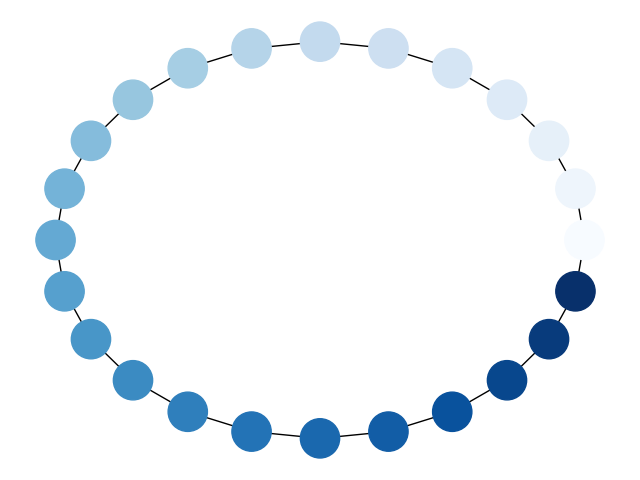# Node Colormap¶

Draw a graph with matplotlib, color by degree.```import matplotlib.pyplot as plt
import networkx as nx

G = nx.cycle_graph(24)
pos = nx.circular_layout(G)
nx.draw(G, pos, node_color=range(24), node_size=800, cmap=plt.cm.Blues)
plt.show()
```

Total running time of the script: ( 0 minutes 0.066 seconds)

Gallery generated by Sphinx-Gallery